• 这个是用Python库画的小猫咪，前面一步步的整个猫的整个身体，具体步骤都写在了注释之中。身体部分的比较快，后面了5个心，由于画心的时候要改变好多度数，而且每次只前进一点点，所以画心的时候会比较慢，...
这个是用Python库画的小猫咪，前面一步步的画整个猫的整个身体，具体步骤都写在了注释之中。身体部分画的比较快，后面画了5个心，由于画心的时候要改变好多度数，而且每次只前进一点点，所以画心的时候会比较慢，大家在看视频的时候会发现这一点。这个东西花了好个小时画出来，好多地方都是几像素几像素的试出来的。不过，我画画能力一般，加上时间有限，并不能完善所有细节。但是我尽可能多的完善了一些线条和布局，弧度之类的的细节，希望大家喜欢我们的作品。希望大家多多支持我们的作品哟！效果图如下：代码如下：from turtle import *#两个函数用于画心def curvemove():for i in range(200):right(1)forward(0.1)def heart(x,y,s):pu()goto(x,y)seth(s)pendown()begin_fill()left(140)forward(11.1)curvemove()left(120)curvemove()forward(11.1)end_fill()#初始化setup(600,600)pu()goto(60,100)pensize(4)pendown()#画左半边的头for i in range(150,212,2):seth(i)fd(3)seth(145)fd(50)left(125)fd(50)for i in range(240,318,2):if i==290:seth(190)fd(10)seth(10)fd(10)elif i==300:seth(200)fd(10)seth(20)fd(10)seth(i)fd(3)#画右半边的头pu()goto(60,100)pendown()seth(45)fd(50)right(125)fd(50)for i in range(-60,-138,-2):if i==-110:seth(-10)fd(10)seth(170)fd(10)elif i==-120:seth(-20)fd(10)seth(160)fd(10)seth(i)fd(3)#头部到这里就画好外观了seth(-40)fd(52)seth(-135)fd(45)pu()seth(-105)fd(5)pendown()fd(17)for i in range(130,106,-3):seth(i)fd(2.5)for i in range(106,30,-10):seth(i)fd(2)seth(38)fd(25)seth(135)fd(31)seth(169)fd(6)seth(270)fd(105)#右边的身子画好了#开始画左边的身子pu()goto(-52,-30)pendown()seth(220)fd(48)#52seth(250)fd(3)seth(270)fd(3)seth(290)fd(2)seth(-40)fd(44)seth(228)fd(20)seth(5)fd(22)#画叉腰的动作pu()goto(-52,-84)seth(133)pendown()fd(22)seth(90)fd(2)seth(60)fd(2)seth(45)fd(29)seth(0)fd(3)seth(-93)fd(102)#叉腰动作结束 接下来画嘴巴 眼睛pu()goto(-43,38)seth(0)pendown()begin_fill()circle(5)end_fill()pu()fd(108)pendown()begin_fill()circle(5)end_fill()#调色环节pu()goto(60,24)pencolor("pink")pensize(6)seth(225)pendown()fd(7)pu()goto(70,24)seth(225)pendown()fd(7)#右半边调色完毕pu()goto(-49,24)seth(225)pendown()fd(7)#画嘴巴pu()pensize(4)pencolor("black")goto(5,21)seth(-45)pendown()fd(5)goto(5,21)seth(225)fd(5)#左边的颜色pu()pencolor("pink")pensize(6)goto(-39,24)seth(225)pendown()fd(7)#给耳朵填充颜色pu()goto(-40,92)seth(80)pendown()fillcolor("pink")begin_fill()circle(14,360,3)end_fill()pu()goto(72,100)seth(-74)pendown()begin_fill()circle(14,360,3)end_fill()#酷酷的黑翅膀pu()pensize(4)color('black', 'black')begin_fill()goto(-90,-35)seth(135)pendown()fd(25)seth(225)fd(45)seth(25)fd(15)seth(-80)pensize(2)fd(15)seth(55)fd(15)seth(25)fd(10)seth(-80)fd(15)seth(75)fd(15)goto(-90,-35)end_fill()#最后一个翅膀pu()goto(125,-30)seth(45)pendown()begin_fill()fd(25)seth(-45)fd(45)seth(155)fd(15)seth(-80)fd(15)seth(120)fd(17)seth(170)fd(15)seth(-80)fd(15)seth(120)fd(17)goto(125,-30)end_fill()#画5颗心speed(10)color('red', 'pink')pensize(2)heart(0,140,0)heart(-125,0,30)heart(140,0,-30)heart(145,-85,-30)heart(-132,-85,30)exitonclick()展开全文• turtle.circle画弧时，海龟（turtle）的方向就是弧的切线方向，也就是说turtle的垂直方向就是圆心在的直线上，给定参数radius就可以画了，程序中第二注意的地方就是小五角星和大五角星的位置关系，主要是程序中的...
• 历时4天，利用工作之余的细碎时间， 修修改改，终于把这...初学turtle时所画的这只柯基，由于对turtle中灵活强大的circle()函数理解和应用不够自如，​​​​​​​自定义的画弧函数化简为繁了。 绘制对象的关键坐...
历时4天，利用工作之余的细碎时间， 修修改改，终于把这只丑萌的小鼓脸柯基画了出来，我也有狗啦~code的过程多坎坷，完成时就有多快乐！成果如下：

谢谢大家给我点了那么多赞，开心鸭~！❥❥❥❥(^_^)

回头来看的小反思小心得：

初学turtle时所画的这只柯基，由于对turtle中灵活强大的circle()函数理解和应用不够自如，​​​​​​​自定义的画弧函数化简为繁了。
绘制对象的关键坐标点确实需要反复尝试，在绘制这样一幅turtle画作时，耐心是远比技术更被需要的。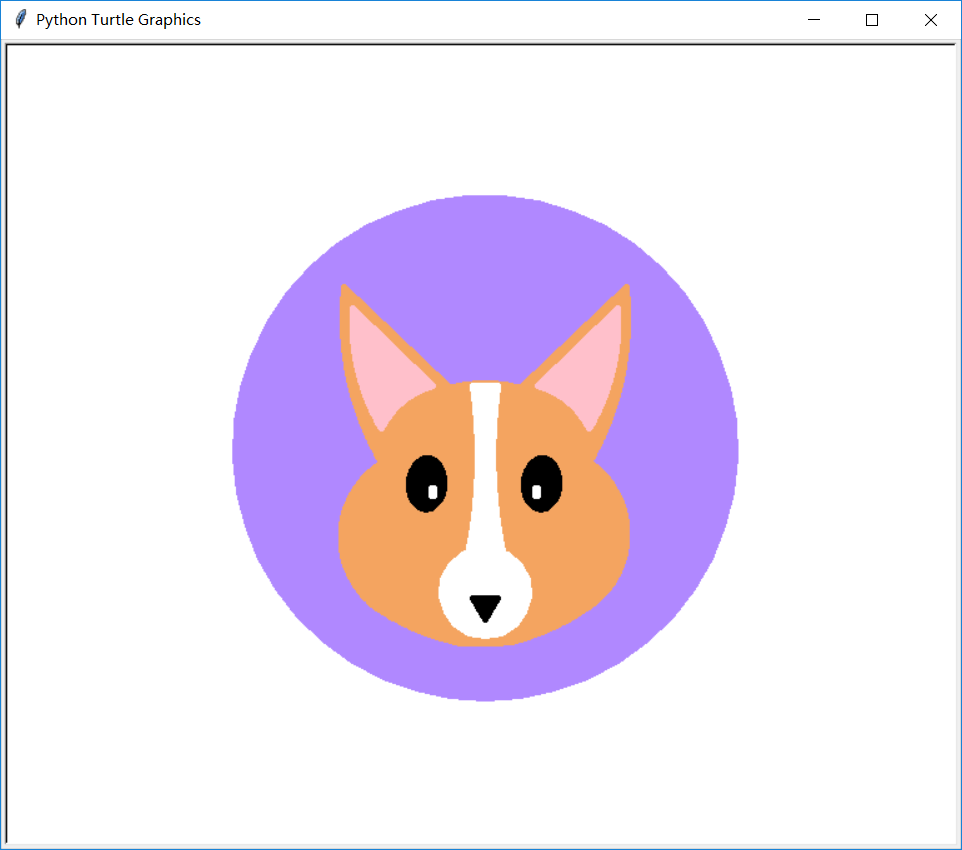绘制柯基时主要用到了以下几种几何图形：

椭圆——柯基眼部（定义函数会更方便，这里可以进一步完善）
弧线——柯基耳部、鼻部、面部（利用"一步一拐曲线绘制法"定义画弧函数）
圆——柯基嘴部白圈（直接利用turtle的circle() 函数）
矩形——柯基眼睛白光块（定义函数）
等边三角形——柯基鼻头（定义函数）
感兴趣的小伙伴请自行了解一下代码：

from turtle import *
pensize(5)
speed(0)
##color('#F4A460')#橘黄
##color('#FFE4E1')#肉粉

##【背景圆】
color('#B088FF')#浅紫
pu()
goto(0,-200)
pd()
begin_fill()
circle(200)
end_fill()

##定义画弧函数
def Arc(initial_degree,range_num,step,rotate_degree):
seth(initial_degree)
for n in range(range_num):
fd(step)
rt(rotate_degree)#

##定义填充矩形函数
def Rect(x,y,height,width):
pu()
goto(x,y)
pd()
begin_fill()
goto(x+width,y)
goto(x+width,y+height)
goto(x,y+height)
goto(x,y)
end_fill()

##定义绘制填充等边三角形函数
def Triangle(x,y,side_length):#等边三角形底边左角
pu()
goto(x,y)
pd()
begin_fill()
seth(0)
fd(side_length)
rt(120)#lt()是正立三角形
fd(side_length)
rt(120)#lt()是正立三角形
fd(side_length)
end_fill()

#中轴线——辅助绘图线
#color("green")
#Rect(-200,0,1,400)#x轴
#Rect(0,-200,400,1)#y轴

##【图层1——面部轮廓】
color('#F4A460')#橘黄
#左耳
pu()
goto(-83.13,-10.94)
pd()
begin_fill()

Arc(120,145,1,1/4)
goto(-30,50)
end_fill()

#右耳
pu()
goto(83.13,-10.94)#(88.13,10.94)
pd()
begin_fill()
Arc(60,145,1,-1/4)
goto(30,50)
end_fill()

#腮帮
#右腮帮
pu()
goto(83.13,-10.94)#0
pd()
begin_fill()
Arc(-35,135,1,9/11)#1
#print(pos())

#下巴
#pencolor("yellow")
Arc(-145,70,1,3/10)#右半下颌2
#print(pos())

#pencolor("red")
Arc(-175,40,1,1/5)#下巴连接线3
#print(pos())

#pencolor("pink")
Arc(168,70,1,3/10)#左半下颌4
#print(pos())

#左腮帮
#pencolor("grey")
Arc(146,135,1,9/11)#5
#print(pos())

#两耳连接
pu()
goto(-30,50)
Arc(15,80,1,1/2)

end_fill()

##【图层2——耳部轮廓】
color('pink')#FFC0CB
#左耳
pu()
goto(-42,50)
pd()
begin_fill()
Arc(-164,55,1,-7/8)
Arc(120,100,1,1/3)
goto(-42,50)
end_fill()

#右耳
pu()
goto(42,50)
pd()
begin_fill()
Arc(-16,55,1,7/8)#(81.13,15.94)
#print(pos())
Arc(60,100,1,-1/3)#(104.15,111.82)
#print(pos())
goto(42,50)
end_fill()

##【图层3——眼部轮廓】
#左黑眼豆豆
pu()
goto(-46,-8)
pd()
color("black")
seth(180)
len = 0.3
begin_fill()
for k in range(2):         # 双弧绘制椭圆
for j in range(60):
if j < 30:
len += 0.04
else:
len -= 0.04

fd(len)
lt(3)
end_fill()
#左眼白光
color("white")
Rect(-43,-38,6,2)

#右黑眼豆豆
pu()
goto(46,-8)
pd()
color("black")
seth(180)
len = 0.3
begin_fill()
for k in range(2):         # 将相同的动作重复做一遍
for j in range(60):
if j < 30:
len += 0.04
else:
len -= 0.04

fd(len)
lt(3)
end_fill()
#右眼白光
color("white")
Rect(40,-38,6,2)

##【图层4——白鼻子轮廓】
pu()
goto(10,50)
pd()
goto(-10,50)
color("white")
begin_fill()
Arc(-82,140,1,1/7)#结束角度A=-82-140*1/7=-102
Arc(-112,20,1.1,-1.2)#结束角度B=-112+20*1.2=-88
#setx(-xcor())
goto(-xcor(),ycor())
seth
Arc(88,20,1.1,-1.2)#求A的y轴对称角度
Arc(102,140,1,1/7)#求8的y轴对称角度
goto(10,50)
end_fill()
pd()

#圆嘴
pu()
goto(0,-150)
seth(0)
pd()
begin_fill()
circle(35)
end_fill()

#黑鼻头
color("black")
Triangle(-10,-120,20)

end_fill()

hideturtle()
done()




展开全文椭圆 曲线
• turtle库中circle()函数用来画弧，但和通常先确定原点，再根据半径、夹角画弧的方法有所不同。使用之后，便能理解circle()函数的巧妙。收获是：边想边做边改胜过完美的空想。 谢谢大家给我点了那么多赞，开心鸭~！...


周末学习了一下turtle库的基本函数，试着画了一只大耳朵小兔子，灵感来源是jellycat邦尼兔。turtle库中circle()函数用来画弧，但和通常先确定原点，再根据半径、夹角画弧的方法有所不同。使用之后，便能理解circle()函数的巧妙。收获是：边想边做边改胜过完美的空想。

谢谢大家给我点了那么多赞，开心鸭~！❥❥❥❥(^_^)

如果想要了解其他turtle库绘画，请点击以下标题：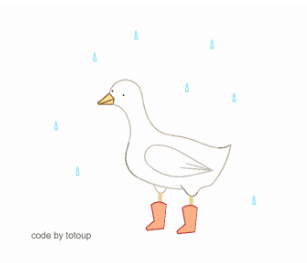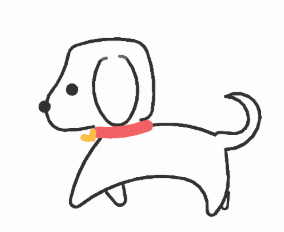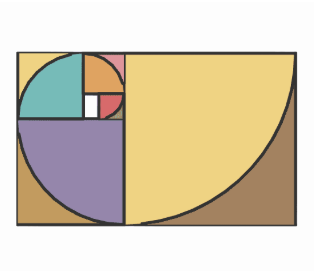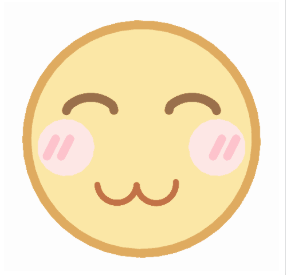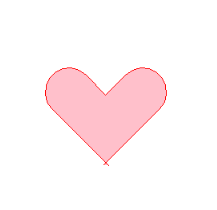Python简笔画——用turtle库画一只《穿红靴子的小鸭》
用Python画一只溜达小狗——turtle库基础入门
如何用Python画一只肥肥的柯基狗狗——turtle库绘制椭圆与弧线实践
用Python绘制斐波那契螺旋线（黄金螺旋线）
用Python画笑脸
用python的turtle库绘制爱心

绘制效果如图 ：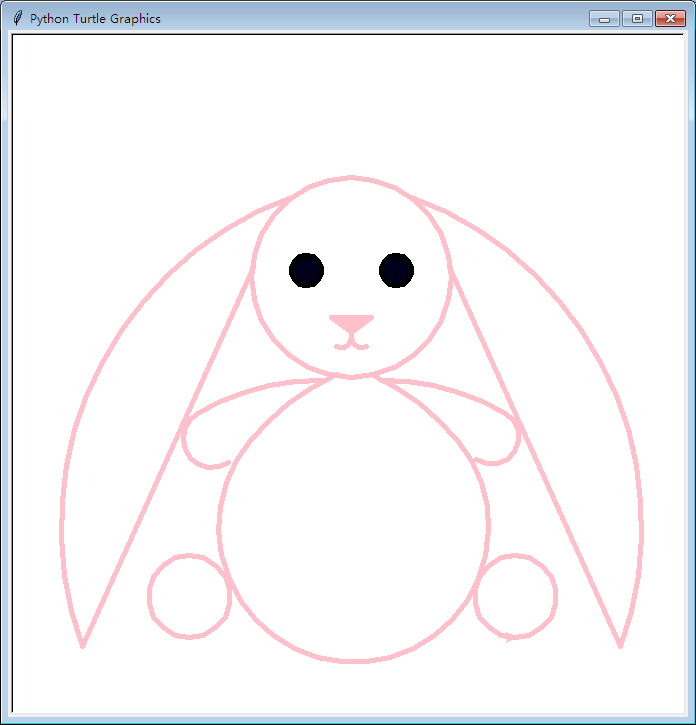在circle(radius,extent)函数中，参数radius取像素值、extent取角度的整数值，两参数均可取正负值。运行以下代码，可以直观地理解circle(radius,extent)函数参数正负值时的绘制特点：

from turtle import *
pensize(5)
pencolor('green')
circle(100,90)

pu()
goto(0,0)
seth(0)
pd()
pencolor('orange')
circle(100,-90)

pu()
goto(0,0)
seth(0)
pd()
pencolor('blue')
circle(-100,90)

pu()
goto(0,0)
seth(0)
pd()
pencolor('red')
circle(-100,-90)


circle()函数以画笔当前方向（y'）为y轴方向，以经过画笔当前绝对坐标（x0,假设y0=0）、垂直于y轴的方向为x轴方向，则圆心（即原点）坐标为（x0-radius=0,0），由当前画笔位置（x0,y0）为弧线起始点，画出extent角度的圆弧。为了方便理解，我绘制了circle()函数的相对坐标体系，如下图。需要注意的是：radius为正时，圆心在当前位置左侧（如下图）；radius为负时，圆心在当前位置右侧；extent为正时，顺画笔当前方向绘制，extent为负时，逆画笔当前方向绘制。

circle()函数可以以画笔当前位置为切点，画出与（画笔当前方向所在直线为）切线相切的任意圆弧。所以在绘制曲线时十分自由好用。抛下【用圆规先确定圆心再画圆】的惯常思维，慢慢使用便能理解circle()函数的巧妙。

以上为个人的学习理解，初识turtle，不当之处欢迎指正。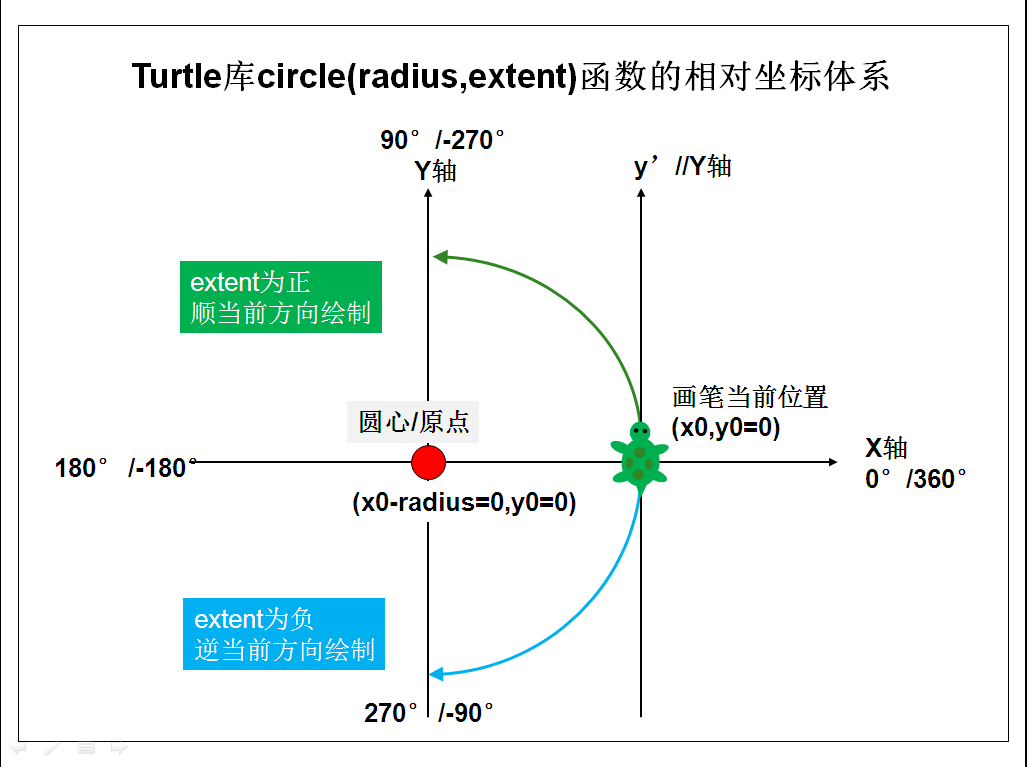兔の绘图代码如下：

原创作品，仅供学习使用，侵权者自重！

#绘制大耳朵兔
from turtle import *
speed(10)

#小兔的面部
color('pink')
pensize(5)
circle(radius=100)#脸

#眼睛
pencolor('black')
#左眼
pu()
goto(-45,92)
pd()
begin_fill()
color((0,0,0),(0,0,0.1))
circle(radius=15)
#右眼
pu()
goto(45,92)
pd()
circle(radius=15)
end_fill()

#鼻子
pu()
goto(20,60)
color('pink')
pd()
begin_fill()
goto(-20,60)
goto(0,45)
goto(20,60)
end_fill()

#嘴
goto(0,45)
goto(0,40)
seth(-90)
circle(10,120)
pu()
goto(0,40)
seth(-90)
pd()
circle(-10,120)

#小兔的耳朵
#左耳
pu()
goto(-60,180)#
seth(200)
pd()
circle(radius=350,extent=90)
goto(-98,110)
#右耳
pu()
goto(60,180)#
seth(-20)
pd()
circle(radius=-350,extent=90)
goto(98,110)

#小兔的身体
pu()
goto(20,3)
seth(-25)
pd()
circle(radius=-250,extent=25)
circle(radius=-135,extent=260)
seth(50)
circle(radius=-250,extent=25)

##小兔的胳膊
#左臂
pu()
seth(180)
goto(-30,-3)
pd()
#小短胳膊
##circle(radius=270,extent=20)
##circle(radius=20,extent=190)
circle(radius=248,extent=30)
circle(radius=29,extent=185)
#右臂
pu()
seth(0)
goto(30,-3)
pd()
circle(radius=-248,extent=30)
circle(radius=-27,extent=184)

##小兔的脚
##左脚
pu()
goto(-162,-260)#
pd()
seth(0)
circle(radius=41)
#右脚
pu()
goto(164,-260)
pd()
circle(radius=41)

done()


展开全文circle
• 这两天在一个公众号里看到好玩的turtle库，今天来学习一下。 turtle.circle(radius, extent=None, steps=None) 描述: 以给定半径画圆 参数: radius(半径); 半径为正(负),表示圆心在画笔的左边(右边)画圆 extent...
• 历时4天，利用工作之余的细碎时间， 修修改改，终于把这只丑萌的...弧线——柯基耳部、鼻部、面部（利用"一步一拐曲线绘制法"定义画弧函数） 圆——柯基嘴部白圈（直接利用turtle的circle() 函数） 矩形——柯基眼睛...
历时4天，利用工作之余的细碎时间， 修修改改，终于把这只丑萌的小鼓脸柯基画了出来，我也有狗啦~code的过程多坎坷，完成时就有多快乐！成果如下：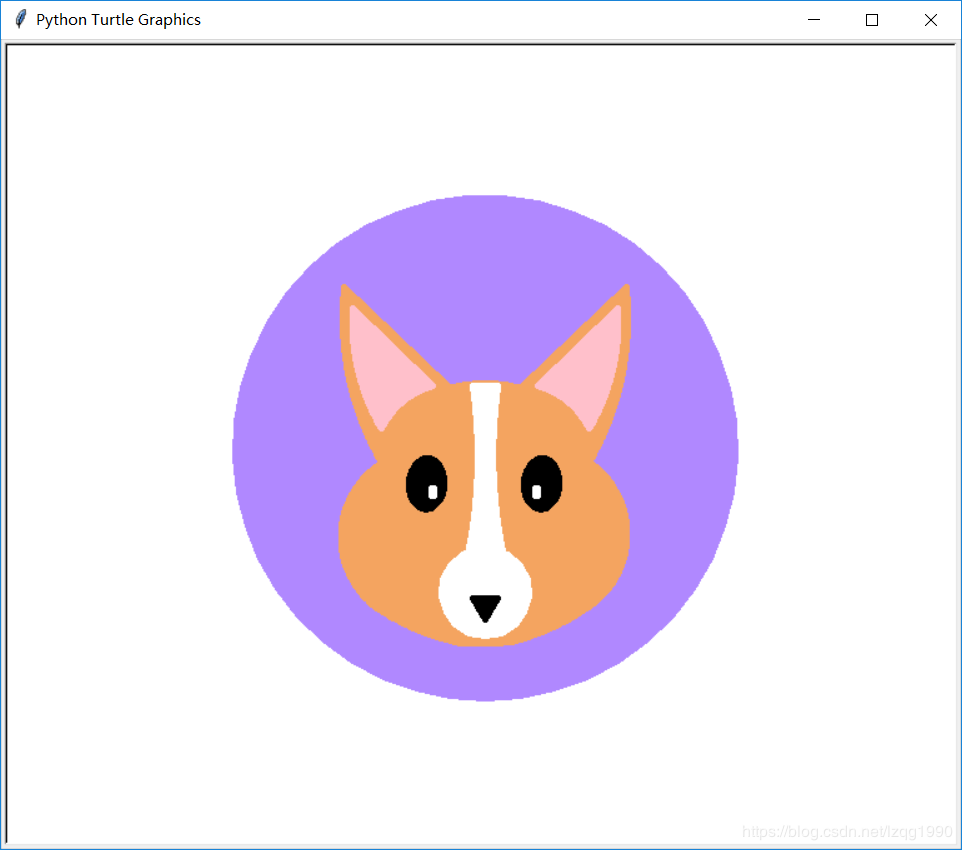绘制柯基时主要用到了以下几种几何图形：
椭圆——柯基眼部（定义函数会更方便，这里可以进一步完善）
弧线——柯基耳部、鼻部、面部（利用"一步一拐曲线绘制法"定义画弧函数）
圆——柯基嘴部白圈（直接利用turtle的circle() 函数）
矩形——柯基眼睛白光块（定义函数）
等边三角形——柯基鼻头（定义函数）
感兴趣的小伙伴请自行了解一下代码：
小编有一个提供免费Python学习资料的圈688244617 快来一起学习吧，还有大量干货哦
from turtle import *
pensize(5)
speed(0)
##color('#F4A460')#橘黄
##color('#FFE4E1')#肉粉

##【背景圆】
color('#B088FF')#浅紫
pu()
goto(0,-200)
pd()
begin_fill()
circle(200)
end_fill()

##定义画弧函数
def Arc(initial_degree,range_num,step,rotate_degree):
seth(initial_degree)
for n in range(range_num):
fd(step)
rt(rotate_degree)#

##定义填充矩形函数
def Rect(x,y,height,width):
pu()
goto(x,y)
pd()
begin_fill()
goto(x+width,y)
goto(x+width,y+height)
goto(x,y+height)
goto(x,y)
end_fill()

##定义绘制填充等边三角形函数
def Triangle(x,y,side_length):#等边三角形底边左角
pu()
goto(x,y)
pd()
begin_fill()
seth(0)
fd(side_length)
rt(120)#lt()是正立三角形
fd(side_length)
rt(120)#lt()是正立三角形
fd(side_length)
end_fill()

#中轴线——辅助绘图线
#color("green")
#Rect(-200,0,1,400)#x轴
#Rect(0,-200,400,1)#y轴

##【图层1——面部轮廓】
color('#F4A460')#橘黄
#左耳
pu()
goto(-83.13,-10.94)
pd()
begin_fill()

Arc(120,145,1,1/4)
goto(-30,50)
end_fill()

#右耳
pu()
goto(83.13,-10.94)#(88.13,10.94)
pd()
begin_fill()
Arc(60,145,1,-1/4)
goto(30,50)
end_fill()

#腮帮
#右腮帮
pu()
goto(83.13,-10.94)#0
pd()
begin_fill()
Arc(-35,135,1,9/11)#1
#print(pos())

#下巴
#pencolor("yellow")
Arc(-145,70,1,3/10)#右半下颌2
#print(pos())

#pencolor("red")
Arc(-175,40,1,1/5)#下巴连接线3
#print(pos())

#pencolor("pink")
Arc(168,70,1,3/10)#左半下颌4
#print(pos())

#左腮帮
#pencolor("grey")
Arc(146,135,1,9/11)#5
#print(pos())

#两耳连接
pu()
goto(-30,50)
Arc(15,80,1,1/2)

end_fill()

##【图层2——耳部轮廓】
color('pink')#FFC0CB
#左耳
pu()
goto(-42,50)
pd()
begin_fill()
Arc(-164,55,1,-7/8)
Arc(120,100,1,1/3)
goto(-42,50)
end_fill()

#右耳
pu()
goto(42,50)
pd()
begin_fill()
Arc(-16,55,1,7/8)#(81.13,15.94)
#print(pos())
Arc(60,100,1,-1/3)#(104.15,111.82)
#print(pos())
goto(42,50)
end_fill()

##【图层3——眼部轮廓】
#左黑眼豆豆
pu()
goto(-46,-8)
pd()
color("black")
seth(180)
len = 0.3
begin_fill()
for k in range(2):         # 双弧绘制椭圆
for j in range(60):
if j < 30:
len += 0.04
else:
len -= 0.04

fd(len)
lt(3)
end_fill()
#左眼白光
color("white")
Rect(-43,-38,6,2)

#右黑眼豆豆
pu()
goto(46,-8)
pd()
color("black")
seth(180)
len = 0.3
begin_fill()
for k in range(2):         # 将相同的动作重复做一遍
for j in range(60):
if j < 30:
len += 0.04
else:
len -= 0.04

fd(len)
lt(3)
end_fill()
#右眼白光
color("white")
Rect(40,-38,6,2)

##【图层4——白鼻子轮廓】
pu()
goto(10,50)
pd()
goto(-10,50)
color("white")
begin_fill()
Arc(-82,140,1,1/7)#结束角度A=-82-140*1/7=-102
Arc(-112,20,1.1,-1.2)#结束角度B=-112+20*1.2=-88
#setx(-xcor())
goto(-xcor(),ycor())
seth
Arc(88,20,1.1,-1.2)#求A的y轴对称角度
Arc(102,140,1,1/7)#求8的y轴对称角度
goto(10,50)
end_fill()
pd()

#圆嘴
pu()
goto(0,-150)
seth(0)
pd()
begin_fill()
circle(35)
end_fill()

#黑鼻头
color("black")
Triangle(-10,-120,20)

end_fill()

hideturtle()
done()

不要错过免费的学习机会 速来  688244617一起交流学习吧
---------------------



展开全文程序员 新手入门
• 1、 导入画笔工具tultle并创建我的画笔:import turtlemypen = turtle.Turtle()由于画圆要用到半径，所以我们给半径定义一个变量radius 并赋值为 1002、 第一个以半径为radius/2，弧度为180的半圆，并开始填充：...
• 前两篇文章基本涵盖了turtle的大部分功能，同时也借由对turtle功能的展示，厘清了Python的一些语法特点，以利于新手入门。但是短短几个例子，阐述得还是有限，这里再展开两个知识点，一方面对turtle做个补遗，另一...
• turtle.circle(radius, extent=None, steps=None) 描述: 以给定半径画圆 参数: radius(半径); 半径为正(负),表示圆心在画笔的左边(右边)画圆 extent(弧度) (optional); steps (optional) (做半径为radius的圆的...
• 利用python-turtle工具，通过调整角度、弧度完成作画，其实用到的方法很简单，就是需要耗费些精力不断地修整，闲话少说，先上个图吧！ 画笔速度较慢，大家耐心等待，也可根据自己习惯调整，直接上代码： import...
• 前两篇文章基本涵盖了turtle的大部分功能，同时也借由对turtle功能的展示，厘清了Python的一些语法特点，以利于新手入门。但是短短几个例子，阐述得还是有限，这里再展开两个知识点，一方面对turtle做个补遗，另一...
• Turtle库是Python内置的图形化模块，属于标准库之一，位于Python安装目录的lib文件夹下，常用函数有以下几种： 画笔控制函数 penup():抬起画笔； pendown():落下画笔； pensize(width):画笔宽度； pencolor...
• 一、圆的另两种用法 1.半圆 在半径的后面加上弧度 import turtle pen = turtle.Turtle() #第一个参数表示半径，第二个参数表示弧度 pen.circle(100,180) turtle.done() 2.内切多边形pycharm
• #turtle.circle(r,extent=None)绘制 默认圆心是左侧r距离的位置，弧度为360 -r右侧 for i in range(4): turtle.circle(40,80) turtle.circle(-40,80) turtle.circle(40,80/2) turtle.fd(40) turtle.circle...
• Turtle（海龟库）1、circle 的三种用法1.1、一个完整的圆1.2、一个不完整的圆1.3、画圆的内切多边形2、更改画布的背景色3、对图形的颜色进行填充 1、circle 的三种用法 circle 有三个参数 第一个参数是半径 第...circle
• 大家好这是我的地一篇博客，我要写一个...import turtle as t t.pensize(2) # 笔大小2像素 t.pencolor("red") # 颜色为红色 t.left(45) # 45度 t.fd(200) # 向前200直线 t.circle(100, 180) # 一圆半径100 弧度180 t
• 下面给大家写的都是书本上的例题 记录一下 第一题： 源代码： from tkinter import * import math WIDTH, HEIGHT = 510, 210 ORIGIN_X, ORIGIN_Y = 2...END_ARC = 360 * 2 #函数图形两个周期 root = Tk() c = Canvas
• 一、 画笔控制函数： 1.penup() 抬起画笔 2.pendown() 落下画笔 3.pensize(width) 调整画笔宽度 ...2.circle(r, extent = None) 在画笔左侧r处为圆心一条弧度为extent的 三、方向控制函数 1.s
• Python turtle标准库的使用简单摸索 turtle绘图窗体 turtle.setup(窗体宽，窗体高，窗体坐标X点，窗体坐标Y点） 画布上，默认有一个坐标原点为画布中心的坐标轴(0,0)，默认"standard"模式坐标原点上有一只面朝x轴...
• Python，感觉你们的都好复杂，那我来个简单的，我是直接把心形看作是一个正方形+两个半圆： 于是这就很简单了，十行代码解决： import turtle as t t.pensize(2) # 笔大小2像素 t.pencolor(red) # 颜色为红色...
• python3.5中已经包括tkinter和turtle模块了，所以想要画图可以直接import turtleturtle中最基本的几个方法： 1、画线： fd(x) #沿着当前方向画x长的线段 ...circle(radius, angle, step) #即可以画圆画弧，也
• 《穿红靴子的小鸭》这幅临摹了ins博主@inky.diary的小鸭水彩，并做了一些调整。 用PythonTurtle库画画，难度不大，熟悉了基础函数后，新手可以快速入门。基础函数可以参考博文《Python标准库之turtle库——...新手入门
• 今天女朋友给我发了一个python的题之前没接触过用tl，题是一个奥运五环，用circle函数来完成。在网上查阅了许多资料,借鉴了很多。 定义：turtle.circle(raidus,extent=None)； 作用：根据半径:white_circle:； ...
• 斐波那契数列(Fibonacci sequence),又称黄金分割数列、因数学家...用python实现的画法是： 以斐波那契数为半径一个90度的扇形，连起来的弧线就是斐波那契螺旋线。 自然界中存在： 艺术作品中也常常出现： ...数学
• turtle库画出美丽的七色彩虹，帮助初学者学习画弧
• 2020/05/05日又是一个无聊的日子，小白初次学习Python绘图，自制机器猫，搞了一下午，瞎，很遗憾，还不会椭圆弧线，画布也不会设置，坐标什么的也没管，直接看着界面了，请大神指教： 绘制效果如下图所示： ...
• turtleturtle.setup()#创建窗体 画笔控制函数 turtle.penup() #海龟起飞 turtle.pendown() #海龟下落 turtle.pensize() #控制轨迹大小 turtle.pencolor() #控制轨迹颜色 运动控制函数 turtle.fd() #海龟...
• 哪个选项不能正确引用turtle库进而使用setup()函数？ import setup from turtle 关于turtle库，哪个选项的描述是错误的？ turtle坐标系的原点默认在屏幕左上角 哪个选项是turtle绘图中角度坐标系的绝对0度方向？...# python画弧turtlepython 订阅